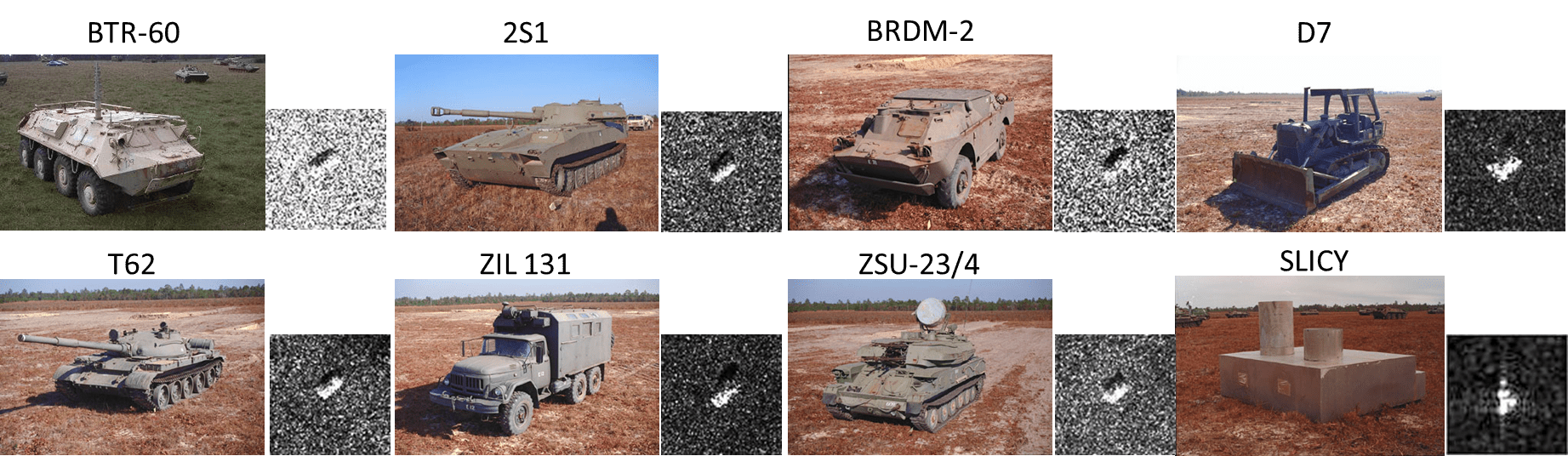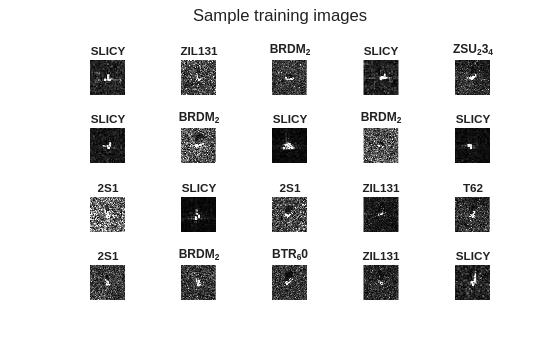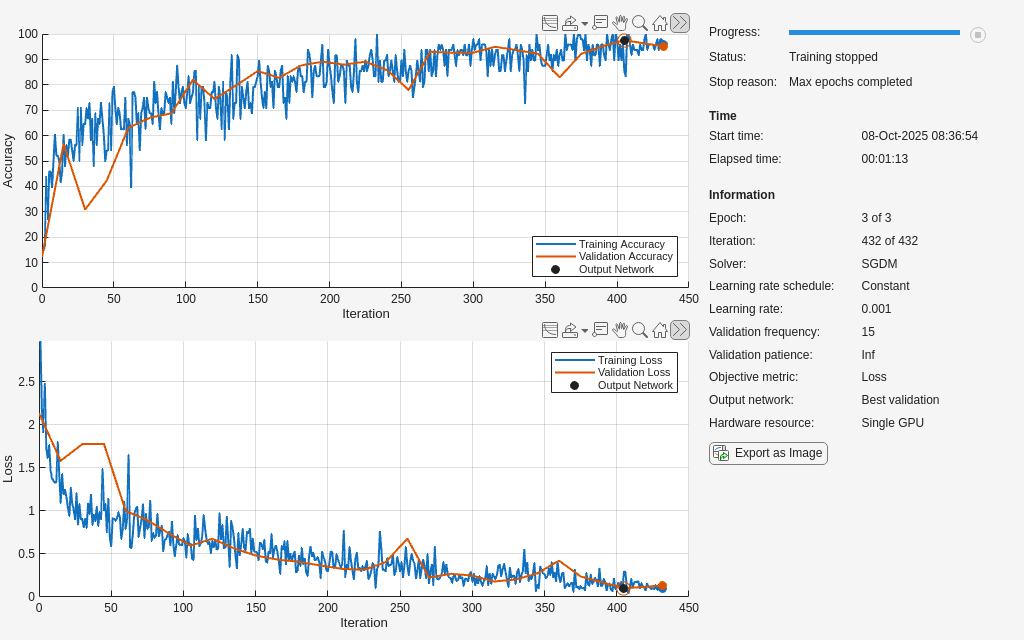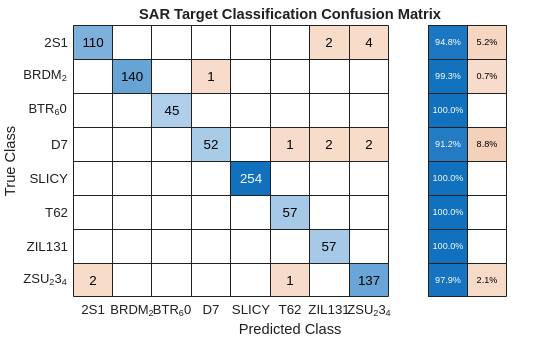# SAR Target Classification using Deep Learning

This example shows how to create and train a simple convolution neural network to classify SAR targets using deep learning.

Deep learning is a powerful technique that can be used to train robust classifier. It has shown its effectiveness in diverse areas ranging from image analysis to natural language processing. These developments have huge potential for SAR data analysis and SAR technology in general, slowly being realized. A major task for SAR-related algorithms has long been object detection and classification, which is called automatic target recognition (ATR). Here we used a simple convolution neural network to train and classify SAR targets using Deep Learning Toolbox™.

The Deep Learning Toolbox provides a framework for designing and implementing deep neural networks with algorithms, pretrained models, and apps.

This example demonstrates how to:

• Load and analyze image data.

• Splitting and augmentation of the data.

• Define the network architecture.

• Train the network.

• Predict the labels of new data and calculate the classification accuracy.

To illustrate this workflow, we will use the Moving and Stationary Target Acquisition and Recognition (MSTAR) Mixed Targets dataset published by the Air Force Research Laboratory . Our goal is to develop a model to classify ground targets based on SAR imagery.

This example uses MSTAR target dataset contains 8688 SAR images from 7 ground vehicle and a calibration target. The data was collected using an X-band sensor in spotlight mode, with a 1-foot resolution. The type of target we used are BMP2 (Infantry Fighting Vehicle), BTR70 (armored car), and T72 (tank). The images were captured at two different depression angles 15 degree and 17 degree with 190 ~ 300 different aspect versions, which are full aspect coverage over 360 degree. Optical images and SAR images of these three types of targets and their replicate targets are shown below in figure.

Download the dataset from the given URL using the `helperDownloadMSTARTargetData` helper function, defined at the end of this example. The size of data set is 28MiB.

```outputFolder = pwd; dataURL = ['https://ssd.mathworks.com/supportfiles/radar/data/' ... 'MSTAR_TargetData.tar.gz']; helperDownloadMSTARTargetData(outputFolder,dataURL);```

### Load and analyze Image Data

Load the SAR image data as an image datastore. `imageDatastore` automatically labels the images based on folder names and stores the data as an `imageDatastore` object. An image datastore enables you to store large image data, including data that does not fit in memory, and efficiently read batches of images during training of a convolutional neural network.

```sarDatasetPath = fullfile(pwd,'Data'); imds = imageDatastore(sarDatasetPath, ... 'IncludeSubfolders',true,'LabelSource','foldernames');```

The MSTAR dataset contains SAR returns from 7 ground vehicles and a calibration target. Optical images and SAR images for these 8 targets are shown belowLet's explore the datastore by randomly displaying some chip images.

```rng(0) figure % Shuffle the datastore. imds = shuffle(imds); for i = 1:20 subplot(4,5,i) img = read(imds); imshow(img) title(imds.Labels(i)) sgtitle('Sample training images') end```The `imds` variable now contains the images and the category labels associated with each image. The labels are automatically assigned from the folder names of the image files. Use `countEachLabel` to summarize the number of images per category.

`labelCount = countEachLabel(imds)`
```labelCount=8×2 table Label Count ________ _____ 2S1 1164 BRDM_2 1415 BTR_60 451 D7 573 SLICY 2539 T62 572 ZIL131 573 ZSU_23_4 1401 ```

First, specify the network input size. When choosing the network input size, consider the memory constraint of your system and the computation cost incured in training.

`imgSize = [128,128,1];`

### Create Datastore Object for Training, Validation and Testing

Divide the data into training, validation and test sets. We will use 80% of our dataset for training, 10% for model validation during training, and 10% for testing after training. `splitEachLabel` splits the datastore `imds` into three new datastores, `imdsTrain`, `imdsValidation`, and `imdsTest`. In doing so, it considers the varying number of images of different classes, so that the training, validation, and test sets have the same proportion of each class.

```trainingPct = 0.8; validationPct = 0.1; [imdsTrain,imdsValidation,imdsTest] = splitEachLabel(imds,... trainingPct,validationPct,'randomize');```

### Data Augmentation

The images in the datastore do not have a consistent size. To train the images with our network, the image size must match the size of the network's input layer. Instead of resizing the images ourselves, we can use an `augmentedImageDatastore`, which will automatically resize the images before passing them into the network. The `augmentedImageDatastore` can also be used to apply transformations, such as rotation, reflection, or scaling, to the input images. This is useful to keep the network from overfitting to our data.

```auimdsTrain = augmentedImageDatastore(imgSize, imdsTrain); auimdsValidation = augmentedImageDatastore(imgSize, imdsValidation); auimdsTest = augmentedImageDatastore(imgSize, imdsTest);```

### Define Network Architecture

Define the convolutional neural network architecture.

`layers = createNetwork(imgSize);`

### Train Network

After defining the network structure, use `trainingOptions` (Deep Learning Toolbox) to specify the training options. Train the network using stochastic gradient descent with momentum (SGDM) with an initial learning rate of 0.001. We set the maximum number of epochs to 3. An epoch is a full training cycle on the entire training data set. Monitor the network accuracy during training by specifying validation data and validation frequency. Shuffle the data every epoch. The software trains the network on the training data and calculates the accuracy on the validation data at regular intervals during training. The validation data is not used to update the network weights. We set `'CheckpointPath'` to a temporary location. This enables the saving of partially trained detectors during the training process. If training is interrupted, such as by a power outage or system failure, you can resume training from the saved checkpoint.

```options = trainingOptions('sgdm', ... 'InitialLearnRate',0.001, ... 'MaxEpochs',3, ... 'Shuffle','every-epoch', ... 'MiniBatchSize',48,... 'ValidationData',auimdsValidation, ... 'ValidationFrequency',15, ... 'Verbose',false, ... 'CheckpointPath',tempdir,... 'Plots','training-progress');```

Train the network using the architecture defined by `layers`, the training data, and the training options. By default, `trainNetwork` uses a GPU if one is available (requires Parallel Computing Toolbox™ and a CUDA® enabled GPU with compute capability 3.0 or higher). For information about the supported compute capabilities, see GPU Support by Release (Parallel Computing Toolbox). Otherwise, it uses a CPU. You can also specify the execution environment by using the `'ExecutionEnvironment'` name-value pair argument of `trainingOptions`.

The training progress plot shows the mini-batch loss and accuracy and the validation loss and accuracy. For more information on the training progress plot, see Monitor Deep Learning Training Progress (Deep Learning Toolbox). The loss is the cross-entropy loss. The accuracy is the percentage of images that the network classifies correctly.

`net = trainNetwork(auimdsTrain,layers,options);`The training process is displayed in the image above. The dark blue line on the upper plot indicates the model's accuracy on the training data, while the black dashed line indicates the model's accuracy on the validation data (separate from training). The validation accuracy is more than 97%, which is quite good for an eight-class classifier. Furthermore, note that the validation accuracy and training accuracy are similar. This indicates that we have a robust classifier. When the training accuracy is much higher than the validation accuracy, the model is overfitting to (i.e. memorizing) the training data.

### Classify Test Images and Compute Accuracy

Predict the labels of the validation data using the trained network and calculate the final accuracy. Accuracy is the fraction of labels that the network predicts correctly.

```YPred = classify(net,auimdsTest); YTest = imdsTest.Labels; accuracy = sum(YPred == YTest)/numel(YTest)```
```accuracy = 0.9827 ```

The test accuracy is very close to the validation accuracy, giving us confidence in the model's predictive ability.

We can use a confusion matrix to study the model's classification behavior in greater detail. A strong center diagonal represents accurate predictions. Ideally, we would like to see small, randomly located values off the diagonal. Large values off the diagonal could indicate specific scenarios where the model struggles.

```figure cm = confusionchart(YPred, YTest); cm.RowSummary = 'row-normalized'; cm.Title = 'SAR Target Classification Confusion Matrix';```Out of the eight classes, the model appears to struggle the most with correctly classifying the ZSU-23/4. The ZSU-23/4 and 2S1 have very similar SAR images and hence we observe some misclassification by our trained model. However, it is still able to achieve greater than 90% accuracy for the class.

### Helper Function

The function `createNetwork` takes as input the image input size `imgSize` and returns a convolution neural network. See below for a description of what each layer type does.

Image Input Layer — An `imageInputLayer` (Deep Learning Toolbox) is where you specify the image size. These numbers correspond to the height, width, and the channel size. The SAR image data consists of grayscale images, so the channel size (color channel) is 1. For a color image, the channel size is 3, corresponding to the RGB values. You do not need to shuffle the data because `trainNetwork`, by default, shuffles the data at the beginning of training. `trainNetwork` can also automatically shuffle the data at the beginning of every epoch during training.

Convolutional Layer — In the convolutional layer, the first argument is `filterSize`, which is the height and width of the filters the training function uses while scanning along the images. In this example, the number 3 indicates that the filter size is 3-by-3. You can specify different sizes for the height and width of the filter. The second argument is the number of filters, `numFilters`, which is the number of neurons that connect to the same region of the input. This parameter determines the number of feature maps. Use the `'Padding'` name-value pair to add padding to the input feature map. For a convolutional layer with a default stride of 1, `'same'` padding ensures that the spatial output size is the same as the input size. You can also define the stride and learning rates for this layer using name-value pair arguments of `convolution2dLayer` (Deep Learning Toolbox).

Batch Normalization Layer — Batch normalization layers normalize the activations and gradients propagating through a network, making network training an easier optimization problem. Use batch normalization layers between convolutional layers and nonlinearities, such as ReLU layers, to speed up network training and reduce the sensitivity to network initialization. Use `batchNormalizationLayer` (Deep Learning Toolbox) to create a batch normalization layer.

ReLU Layer — The batch normalization layer is followed by a nonlinear activation function. The most common activation function is the rectified linear unit (ReLU). Use `reluLayer` (Deep Learning Toolbox) to create a ReLU layer.

Max Pooling Layer — Convolutional layers (with activation functions) are sometimes followed by a down-sampling operation that reduces the spatial size of the feature map and removes redundant spatial information. Down-sampling makes it possible to increase the number of filters in deeper convolutional layers without increasing the required amount of computation per layer. One way of down-sampling is using a max pooling, which you create using `maxPooling2dLayer` (Deep Learning Toolbox). The max pooling layer returns the maximum values of rectangular regions of inputs, specified by the first argument, `poolSize`. In this example, the size of the rectangular region is [2,2]. The `'Stride'` name-value pair argument specifies the step size that the training function takes as it scans along the input.

Fully Connected Layer — The convolutional and down-sampling layers are followed by one or more fully connected layers. As its name suggests, a fully connected layer is a layer in which the neurons connect to all the neurons in the preceding layer. This layer combines all the features learned by the previous layers across the image to identify the larger patterns. The last fully connected layer combines the features to classify the images. Therefore, the `OutputSize` parameter in the last fully connected layer is equal to the number of classes in the target data. In this example, the output size is 10, corresponding to the 10 classes. Use `fullyConnectedLayer` (Deep Learning Toolbox) to create a fully connected layer.

Softmax Layer — The softmax activation function normalizes the output of the fully connected layer. The output of the softmax layer consists of positive numbers that sum to one, which can then be used as classification probabilities by the classification layer. Create a softmax layer using the `softmaxLayer` (Deep Learning Toolbox) function after the last fully connected layer.

Classification Layer — The final layer is the classification layer. This layer uses the probabilities returned by the softmax activation function for each input to assign the input to one of the mutually exclusive classes and compute the loss. To create a classification layer, use `classificationLayer` (Deep Learning Toolbox).

```function layers = createNetwork(imgSize) layers = [ imageInputLayer([imgSize(1) imgSize(2) 1]) % Input Layer convolution2dLayer(3,32,'Padding','same') % Convolution Layer reluLayer % Relu Layer convolution2dLayer(3,32,'Padding','same') batchNormalizationLayer % Batch normalization Layer reluLayer maxPooling2dLayer(2,'Stride',2) % Max Pooling Layer convolution2dLayer(3,64,'Padding','same') reluLayer convolution2dLayer(3,64,'Padding','same') batchNormalizationLayer reluLayer maxPooling2dLayer(2,'Stride',2) convolution2dLayer(3,128,'Padding','same') reluLayer convolution2dLayer(3,128,'Padding','same') batchNormalizationLayer reluLayer maxPooling2dLayer(2,'Stride',2) convolution2dLayer(3,256,'Padding','same') reluLayer convolution2dLayer(3,256,'Padding','same') batchNormalizationLayer reluLayer maxPooling2dLayer(2,'Stride',2) convolution2dLayer(6,512) reluLayer dropoutLayer(0.5) % Dropout Layer fullyConnectedLayer(512) % Fully connected Layer. reluLayer fullyConnectedLayer(8) softmaxLayer % Softmax Layer classificationLayer % Classification Layer ]; end function helperDownloadMSTARTargetData(outputFolder,DataURL) % Download the data set from the given URL to the output folder. radarDataTarFile = fullfile(outputFolder,'MSTAR_TargetData.tar.gz'); if ~exist(radarDataTarFile,'file') disp('Downloading MSTAR Target data (28 MiB)...'); websave(radarDataTarFile,DataURL); untar(radarDataTarFile,outputFolder); end end```

#### References

 MSTAR Dataset. https://www.sdms.afrl.af.mil/index.php?collection=mstar